Surface of the form: (F(x,y,z) = constant)

In:=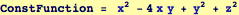Out=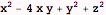In:=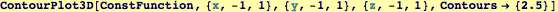Out=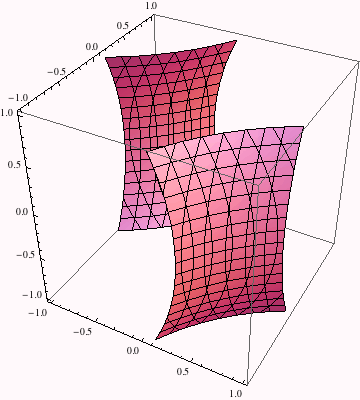The following statements produce contour plots of the same function, using two different methods for colorizing the surfaces...

In:=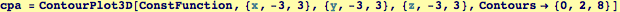Out=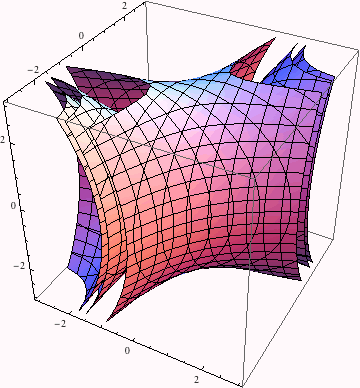In:=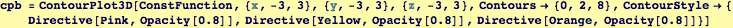Out=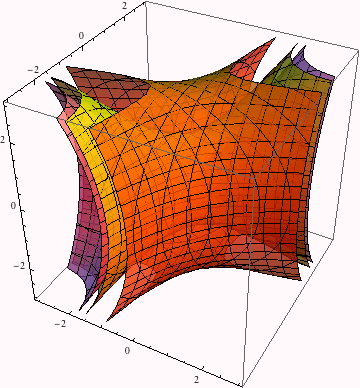In:=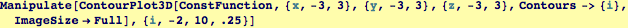Out=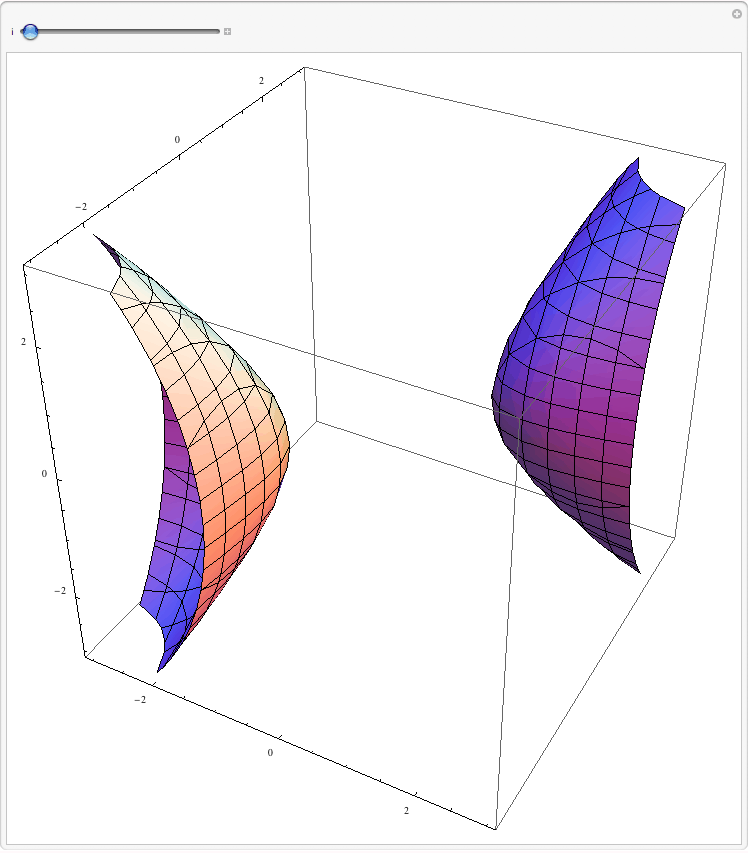Created by Wolfram Mathematica 6.0  (28 September 2007)